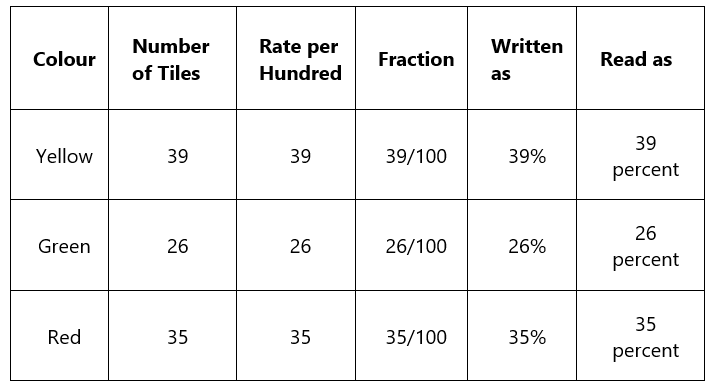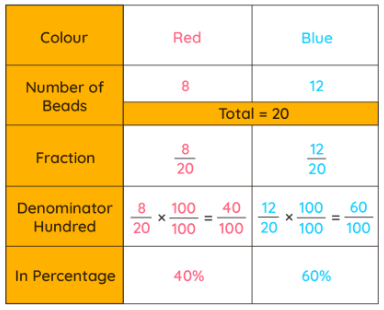JAMB  >  Percentage

# Percentage - Notes | Study Mathematics for JAMB - JAMB

 1 Crore+ students have signed up on EduRev. Have you?

The term "percentage" was adapted from the Latin word "per centum", which means "by the hundred". Percentages are fractions with 100 as the denominator. In other words, it is the relation between part and whole where the value of whole is always taken as 100.

What is Percentage?

Percentage is a fraction or a ratio in which the value of whole is always 100. For example, if Sam scored 30% marks in his math test, it means that he scored 30 marks out of 100. It is written as 30/100 in the fraction form and 30:100 in terms of ratio.
Percentage Definition: Percentage is defined as a given part or amount in every hundred. It is a fraction with 100 as the denominator and is represented by the symbol "%".

Calculation of Percentage

Calculating percentage means to find the share of a whole, in terms of 100. There are two ways to find a percentage:

• By using the unitary method.
• By changing the denominator of the fraction to 100.

It should be noted that the second method for calculating percentage is not used in situations where the denominator is not a factor of 100. For such cases we use the unitary method.

How to get a Percentage?

Percent is another name for indicating hundredths. Thus, 1% is one-hundredth, that means 1%=1/100=0.01.
Let's calculate percentage using the two methods given above.
When we have two or more values that add up to 100, then the percentage of those individual values to the total value is that number itself. For example, Sally bought tiles of three different colors for her house. The details of the purchase are given in the following table.Since the total number of items adds up to 100, the percentages can be easily calculated.
What if the total number of items do not add up to 100? In such cases, we convert the fractions to equivalent fractions with the denominator as 100. For example, Emma has a bracelet which is made up of 20 beads of two different colours, red and blue. Observe the following table which shows the percentage of red and blue beads out of the 20 beads.Emma's sisters, Nora and Jenny, calculated the percentages as well, but in different ways.
Nora used the unitary method. Using the unitary method for calculating percentage, we say that out of 20 beads, the number of red beads are 8. Hence, out of 100, the number of red beads will be 8/20 × 100= 40%.
Jenny converted the fraction 8/20 into an equivalent fraction 40/100 by multiplying the numerator and denominator with 5/5.
So, 8/20 = (8 × 5)/(20 × 5)
= 40/100
= 40%

Formula to Calculate Percentage

The percentage formula is used to find the share of a whole in terms of 100. Using this formula, you can represent a number as a fraction of 100. If you observe carefully, all the three ways to get percentage shown above can be easily calculated by using the formula given below:
Percentage = (Value/Total Value) × 100

Percentage Difference Between Two Numbers

Percentage difference is the change in the value of a quantity over a period of time in terms of percentage. Sometimes we need to know the increase or decrease in some quantity as percentages, which is also referred to as Percentage Change. For example, an increase in population, a decrease in poverty, and so on.
We have the formula to show the change in quantity as a percentage. There are two cases that might arise while calculating percentage difference and those are:

• Calculate percentage increase
• Calculate percentage decrease

How to Calculate Percentage Increase?

Percentage increase refers to the perchange change in the value when it is increased over a period of time. For example, population increase, increase in the number of bacteria on a surface, etc. Percentage increase can be calculated by using the following formula:
Percentage Increase= (Increased Value-Original value)/Original value × 100

How to Calculate Percentage Decrease?

Percentage decrease refers to the perchange change in the value when it is decreased over a period of time. For example, decrease in the level of rainfall, decrease in the number of Covid patients, etc. Percentage decrease can be calculated by using the following formula:
Percentage Decrease= (Original value-Decreased Value)/Original Value × 100

Points to Remember:

• To find the percentage of a whole, work out the value of 1% and then multiply it by the percent we need to find.
• An increase or decrease in any quantity can be expressed as a percentage.
• Fractions can be converted into percentages and vice-versa.
• Percentages are reversible. For example, 25% of 40 is the same as 40% of 25.
The document Percentage - Notes | Study Mathematics for JAMB - JAMB is a part of the JAMB Course Mathematics for JAMB.
All you need of JAMB at this link: JAMB

## Mathematics for JAMB

35 videos|17 docs|13 tests
 Use Code STAYHOME200 and get INR 200 additional OFF

## Mathematics for JAMB

35 videos|17 docs|13 tests

Track your progress, build streaks, highlight & save important lessons and more!

,

,

,

,

,

,

,

,

,

,

,

,

,

,

,

,

,

,

,

,

,

;